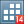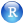Germán Rodríguez
Generalized Linear Models Princeton University

## Robust Standard Errors in R

Stata makes the calculation of robust standard errors easy via the `vce(robust)` option. Replicating the results in R is not exactly trivial, but Stack Exchange provides a solution, see replicating Stata’s robust option in R.So here’s our final model for the program effort data using the `vce(robust)` option in Stata:

```. use https://grodri.github.io/datasets/effort, clear
(Family Planning Effort Data)

. recode effort (min/4=1 "Weak") (5/14=2 "Moderate") (15/max=3 "Strong"), gen(effortg)
(20 differences between effort and effortg)

. regress change setting i.effortg, vce(robust)

Linear regression                               Number of obs     =         20
F(3, 16)          =      28.29
Prob > F          =     0.0000
R-squared         =     0.8016
Root MSE          =      5.732

─────────────┬────────────────────────────────────────────────────────────────
│               Robust
change │ Coefficient  std. err.      t    P>|t|     [95% conf. interval]
─────────────┼────────────────────────────────────────────────────────────────
setting │   .1692677   .0454318     3.73   0.002     .0729565    .2655788
│
effortg │
Moderate  │   4.143915    3.19122     1.30   0.213     -2.62117      10.909
Strong  │   19.44761   2.567472     7.57   0.000     14.00481    24.89041
│
_cons │  -5.954036   2.707697    -2.20   0.043     -11.6941   -.2139743
─────────────┴────────────────────────────────────────────────────────────────
```Here’s how to get the same result in R. Basically you need the `sandwich` package, which computes robust covariance matrix estimators. You also need some way to use the variance estimator in a linear model, and the `lmtest` package is the solution. You will not get the same results as Stata, however, unless you use the `HC1` estimator; the default is `HC3`, for reasons explained in `?vcovHC`.

```> library(haven)
> fpe\$effortg = cut(fpe\$effort,  breaks=c(min(fpe\$effort),5,15,max(fpe\$effort)),
+   right=FALSE, include.lowest=TRUE, labels=c("Weak","Moderate","Strong"))
> m <- lm(change ~ setting + effortg, data = fpe)
> library(lmtest)
> library(sandwich)
> coeftest(m, vcov = vcovHC(m, type="HC1"))

t test of coefficients:

Estimate Std. Error t value  Pr(>|t|)
(Intercept)     -5.954036   2.707697 -2.1989   0.04294 *
setting          0.169268   0.045432  3.7258   0.00184 **
effortgModerate  4.143915   3.191220  1.2985   0.21251
effortgStrong   19.447609   2.567472  7.5746 1.118e-06 ***
---
Signif. codes:  0 '***' 0.001 '**' 0.01 '*' 0.05 '.' 0.1 ' ' 1
```

The main point is that the results are exactly the same. Interestingly, some of the robust standard errors are smaller than the model-based errors, and the effect of setting is now significant.